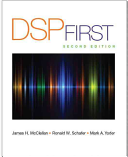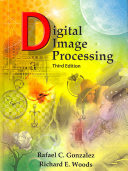### Information Processing

Data is displayed for academic year: 2022./2023.

#### Course Description

The course will provide basic insight into information processing techniques, accompanied by overviews of several applications and their respective information processing tools. Students will learn about time analysis, frequency analysis and time-frequency analysis of signals. Basic techniques from machine learning will be presented, with special attention to artificial neural networks. Selected application areas include: speech processing and analysis, image processing and analysis, bioinformatics, and computational finance.

#### Study Programmes

[FER3-EN] Computing - study
Elective Courses (5. semester)
[FER3-EN] Electrical Engineering and Information Technology - study
Elective Courses (5. semester)

#### Learning Outcomes

1. Identify and explain fundamental techniques of signal processing
2. Identify and explain fundamental techniques of machine learning
3. Identify and explain fundamental techniques of image processing analysis
4. Identify and explain fundamental techniques of text analysis
5. Identify and explain fundamental techniques of speech analysis
6. Identify and explain fundamental techniques of computational finance
7. Identify and explain fundamental techniques of bioinformatics
8. Identify and explain fundamental techniques of complex networks

#### Forms of Teaching

Lectures

Lectures take place in a lecture room with a lecturer who is an expert on a specific subject. The lecture is interactive, and students are expected to participate in the discussion.

Laboratory

Laboratory exercises are held in labs equipped with computers. During the exercise, students try out theoretical concepts and apply them to specific problems

Continuous Assessment Exam
Laboratory Exercises 50 % 18 % 50 % 18 %
Mid Term Exam: Written 50 % 40 % 0 %
Final Exam: Written 50 % 42 %
Exam: Written 50 % 82 %

#### Week by Week Schedule

1. Introduction. Basic concepts.
2. Continuous time Fourier series. Introduction to signal processing in Python. Application to river water level.
3. Discrete Fourier transform. Short-time Fourier transform. Wavelet transform. Application to river water level.
4. Introduction to Bioinformatics. Data types in bioinformatics. Alignment algorithms.
5. Coding DNA sequences as signal. Signal convolution as a measure of similarity. MAFFT algorithm, determining sequence similarity using Fast Fourier Transform.
6. Hidden Markov models (HMM) and their application for time series modeling; Models with discrete observations. Evaluation of the likelihood of a series of observations for a given model (forward algorithm and backward algorithm).
7. Hidden Markov models (HMM) - finding the most probable sequence of hidden states (Viterbi algorithm); Determining the optimal parameters of the HMM model from multiple observation sequences (model training).
8. Midterm exam
9. Introduction to quantitative finance. Data types in finance. Basic properties of financial time series.
10. Introduction to multivariate data analysis. Principal component analysis. Applications in quantitative finance.
11. Digital image analysis. Problem of object detection. Fundamentals of functioning and training of artificial neural networks.
12. Peculiarities and practical aspects of the neural network training procedure. Classification of input samples. Detection of objects by neural networks.
13. Data, signals, systems, invariants and symmetries. Fringe projection profilometry.
14. Registration and iterative closest point algorithm (ICP).
15. Final exam

#### LiteratureJames H. McClellan, Ronald Schafer, Mark Yoder (2015.), Signal Processing First, Pearson College DivisionRafael C. Gonzalez, Richard Eugene Woods (2008.), Digital Image Processing, Prentice Hall

#### General

ID 223365
Winter semester
5 ECTS
L1 English Level
L1 e-Learning
45 Lectures
0 Seminar
0 Exercises
15 Laboratory exercises
0 Project laboratory